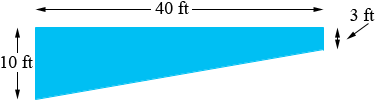SEARCH HOMEMath Central Quandaries & QueriesQuestion from Ron, a student: What is the volume of a swimming pool when its length is 40 ft, width 20ft, the deep end is 10 ft and the shallow end is 3 ft.?Hi Ron,

The volume of the pool is the area of the cross-section times the width of 20 feet. If the bottom of the pool is a gradual slope from 3 feet to 10 feet then the cross-section is a trapezoid.The area of a trapezoid is the average of the lengths of the parallel sides times the distance between the parallel sides. In this case the average of the length of the parallel sides is (10 + 3)/2 feet and the distance between the parallel sides is 40 feet.

I hope this helps,
PennyMath Central is supported by the University of Regina and The Pacific Institute for the Mathematical Sciences.